# Inductor Coupling - Series & Parallel Combinations

Published  April 15, 2019   0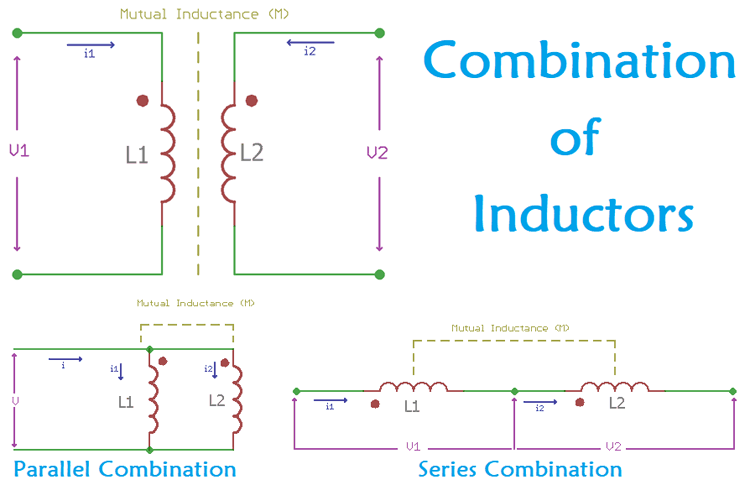In previous tutorial, we started with Understanding an Inductor and It's Working, now it is time to explore the different combinations of Inductors. In electronics, Inductors are the most commonly used components after the capacitors and resistors, which are used in different combinations for different applications. We have also used inductor to build metal detectors and measured the value of inductor using different techniques, all the links are given below:

### What is coupled circuits?

The combinations of components are together to create coupled circuits. The meaning of coupled circuit is that the energy transfer takes place from one to other when either of the circuits is energized. Major components in the electronics circuit are coupled by either conductively or electromagnetically.

However, in this tutorial, the electromagnetic coupling and the combination of inductors, like inductors in series or parallel combinations will be discussed.

### Mutual Inductance

In the previous article, we discussed the self-inductance of an inductor and its parameter. During the self-inductance related operation, there was no mutual inductance took place.

When the rate of current change occurs, a voltage is induced inside a coil. Which can be further demonstrated using the below formula where,

V(t) is the induced voltage inside the coil, i Is the current flowing through the coil, and the inductance of the coil is L.

```V(t) = L {di(t)/dt}
```

The above condition is true only for the self-inductance related circuit element where two terminals are present. In such a case, no mutual inductance is taken into the order.

Now, at the same scenario, if two coils are situated in a close distance, the inductive coupling will happen.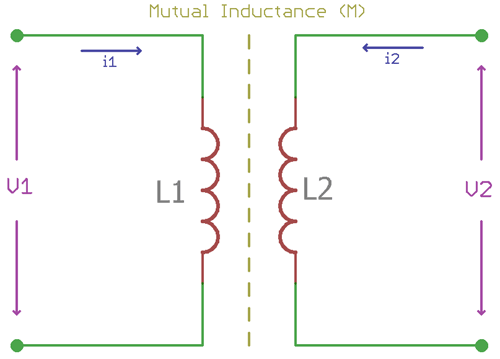In the above image, two coils are shown. These two coils are very close to each other. Due to the current i1 flowing through the coil L1, magnetic flux is induced which will then get transferred to the other coil L2.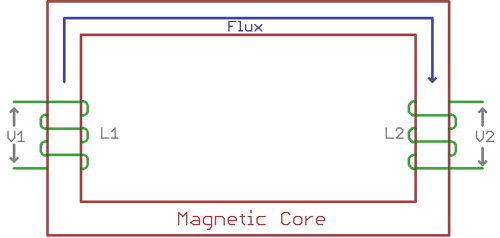In the above image, the same circuit is now tightly wrapped in a core material so that the coils cannot move. As the material is a magnetic core, it has permeability. The two separate coils are now magnetically coupled. Now, interestingly, if one of the coils faces the rate of current change, the other coil will induce a voltage which is directly proportional to the rate of current change in the other coil.

Therefore, when a voltage source V1 is applied in the coil L1, the current i1 will start to flow through the L1. The rate of current change produces a flux which flows through the magnetic core and produces a voltage in the coil L2. The rate of current change in L1 also changes the flux which can further manipulate the induced voltage in L2.

The induced voltage in the L2 can be calculated in the below formula-

```V2 = M {di1(t)/dt}
```

In the above equation, there is an unknown entity. That is M. This is because, mutual inductances are responsible for the mutually induced voltage in two independent circuits. This M, mutual inductance is the coefficient proportionality.

Same for the first coil L1, the mutually induced voltage due to mutual inductance for the first coil can be –

`V2 = M {di2(t)/dt}`

Same as like the inductance, mutual inductance is also measured in Henry. The maximum value of mutual inductance can be √L1L2. As the inductance induces voltage with the rate of current change, mutual inductance also induces a voltage, which is termed as mutual voltage M(di/dt). This mutual voltage can be positive or negative which is highly dependable on the physical construction of the coil and the direction of the current.

### DOT Convention

The Dot Convention is an essential tool to determine the polarity of the mutually induced voltage. As the name suggests, the dot mark which is in a circular shape is a special symbol which is used at the end of two coils in mutually coupled circuits. This dot also provides the information of the winding construction around its magnetic core.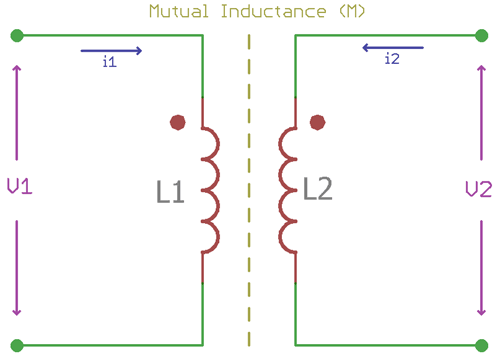In the above circuit, two mutually coupled inductors are shown. These two inductors have self-inductances of L1 and L2.

The voltages V1 and V2 are developed across the inductors are the result of current entering into the inductors on the dotted terminals. By assuming that the mutual inductance of those two inductors is M, The induced voltage can be calculated using the below formula,

For the first inductor L1, the induced voltage will be -

`V1 = L1(di1/dt) ± M(di2/dt)`

The same formula can be used for calculating the induced voltage of the second Inductor,

`V2 = L2(di2/dt) ± M(di1/dt)`

Therefore, the circuit contains two types of induced voltage, the induced voltage due to self-inductance and the mutually induced voltage due to the mutual inductance. The induced voltage depending on the self-inductance is calculated using the formula V = L(di/dt) which is positive, but the mutually induced voltage can be negative or positive depending on the winding construction as well as the flow of current. The use of dot is an important parameter to determine the polarity of this mutually induced voltage.

In a coupled circuit where two terminals belong two different coils and identically marked with dots, then for the same direction of the current which is relative to like terminals, the magnetic flux of self and mutual induction in each coil will add up together.

### Coefficient of Coupling

The coefficient of inductor coupling is an important parameter for coupled circuits to determine the amount of coupling between the inductively coupled coils. The coefficient of coupling is expressed by the letter K.

The formula of the coefficient of coupling is K = M/√L1+L2 where L1 is the self inductance of the first coil and the L2 is the self inductance of the second coil.

Two inductively coupled circuits are linked using the magnetic flux. If the entire flux of one inductor is coupled or linked the other inductor is called perfect coupling. During this situation, the K can be expressed as 1 which is the short form of 100% coupling. The coefficient of coupling will always less than the unity and the maximum value of the coefficient of coupling can be 1 or 100%.

The mutual inductance is highly dependable on the coefficient of coupling between the two inductively coupled coil circuits. If the coefficient of coupling is higher so the mutual inductance will be higher, on the other side, if the coefficient of coupling is at a lower amount that will highly decrease the mutual inductance in the coupling circuit. The coupling coefficient cannot be a negative number and it has no dependencies on the direction of current inside the coils. The coupling coefficient depends on the core materials. In iron or ferrite core materials the coupling coefficient can be very high like 0.99 and for the air core, it can be as low as 0.4 to 0.8 depending on the space between the two coils.

### Inductor in Series Combination

Inductors can be added together in series. There are two ways to connect inductors in series, by using Aiding Method or by using Opposition Method.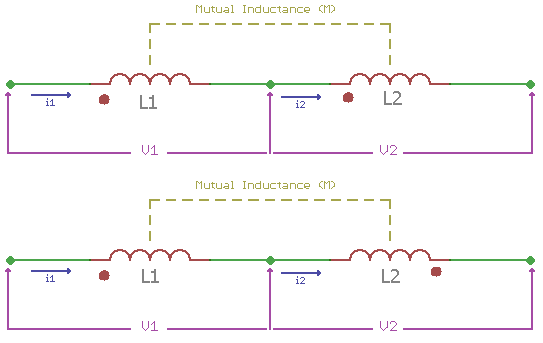In the above image, two types of series connections are shown. For the first one on the left side, the inductors are connected in series by Aiding Method. In this method, the current flowing through the two inductors is in the same direction. As the current flowing in the same direction, the magnetic fluxes of self and mutual induction will end up linking with each other and add together.

Therefore, the total inductance can be calculated using the below formula-

`Leq = L1 +L2 +2M`

Where, Leq is the total equivalent inductance and M is the mutual inductance.

For the right image, the Opposition Connection is shown. In such a case, the current flow through the inductors is in the opposite direction. Therefore, the total inductance can be calculated using the below formula,

`Leq = L1 +L2 - 2M`

Where, Leq is the total equivalent inductance and M is the mutual inductance.

### Inductors in Parallel Combination

Same as the series inductor combination, the parallel combination of two inductors can be two types, by using aiding method and by using opposition method.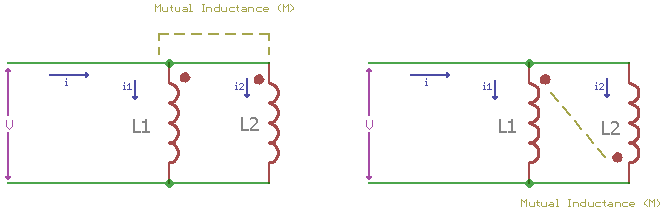For the Aiding Method, as seen on the left image, the dot convention clearly shows that the current flow through the inductors is in the same direction. To calculate the total inductance, below formula can be very helpful. In such a case, the self-induced electromagnetic field in two coils allows the mutually induced emf.

`Leq = (L1L2 – M2) / (L1 +L2 +2M)`

For the Opposition Method, the inductors are connected in parallel with the opposite direction of each other. In such a case, the mutual inductance creates a voltage which opposes the self-induced EMF. The equivalent inductance of the parallel circuit can be calculated using the below formula-

`Leq = (L1L2 – M2) / (L1 +L2 +2M)`

### Applications of Inductor

One of the best usage of coupled inductors is in the creation of transformers. A transformer uses coupled inductors wrapped around iron or ferrite core. An ideal transformer has zero loss and hundred percent coupling coefficients. Other than the transformer, coupled inductors are also used in sepic or flyback converter. This is an excellent choice to isolate the primary input with the secondary output of power supply by using the coupled inductor or transformers.

Apart from that coupled inductors are also used to make a single or double tuned circuit in radio transmitting or receiving circuit